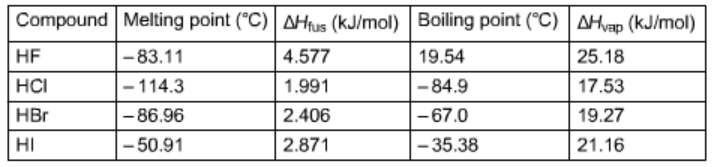# Problem: Using the following data, Calculate ΔSfus and Δvap for HBr.Determine the entropy change when 4.80 mol of HBr (f) freezes at atmospheric pressure.

###### FREE Expert Solution

Using the following data,Calculate ΔSfus:

89% (288 ratings)###### Problem Details

Using the following data,Calculate ΔSfus and Δvap for HBr.

Determine the entropy change when 4.80 mol of HBr (f) freezes at atmospheric pressure.